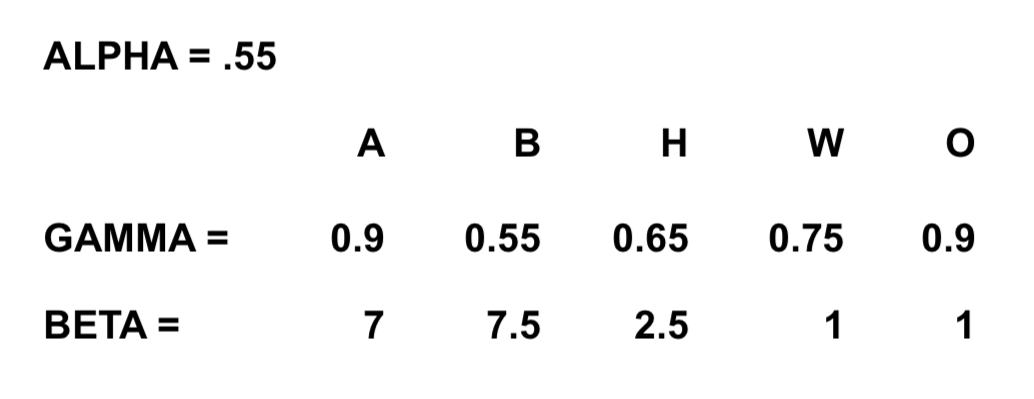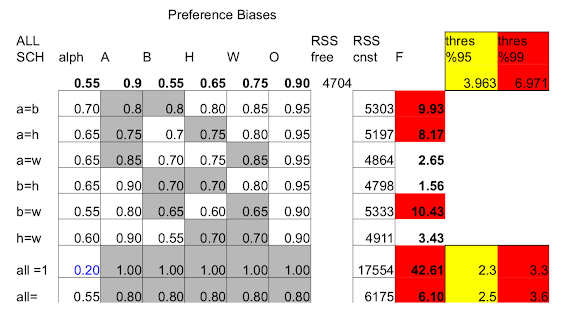# Hybrid Network Models¶

• Most networks involve both choice and chance in formation
• What are the relative roles?
• Random/Strategic models can be too extreme
• Can we see relative roles in homophily?

# Application - Homophily¶

• Group A and Group B form fewer cross race friendships than would be expected given population mix
• Is it due to structure: few meetings?
• Is it due to preferences of group A?
• Is it due to preferences of group B?

# Currarini, Jackson, Pin(09,10)¶

• Utilities specified as a function of friendships
• Meeting process that incorporates randomness
• Allow both utilities and meeting process to depend on types

# Revealed Preference Theory¶

• Common to Consumer Theory
• Use it in mapping social/friendship choices to!
• Different information than surveys on racial attitudes

# Model¶

Types: $i \in \{1,...,k\}$

$s_i$ = # same-type friends $d_i$ = # different-type friends

$U_i = (s_i + \gamma_i d_i)^{\alpha}$ utility to type i

• $\gamma_i$ is the preference bias
• $\alpha < 1$ captures diminishing returns

# Individual Choice¶

• $t_i$ number of friends -- proportional to time spent socializing -- i is "type"

• $q_i$ fraction of friends that will be of own type

• $t_i$ maximize $(q_it_i + \gamma_i (1-q_i)t_i)^\alpha - ct_i$

• Solution: $t_i = (\alpha /c)^{1/(1-\alpha)}(q_i + \gamma_i(1 - q_i))^{\alpha/(1-\alpha)}$
• Add noise for particular agent a of type i: $t_{ai} = (\alpha /c)^{1/(1-\alpha)}(q_i + \gamma_i(1 - q_i))^{\alpha/(1-\alpha)} + \epsilon_\alpha$

# How to identify preference parameters from data?¶

• $t_{ai} = (\alpha /c)^{1/(1-\alpha)}(q_i + \gamma_i(1 - q_i))^{\alpha/(1-\alpha)} + \epsilon_\alpha$

• This is observed directly in the data and will vary with $q_i$

• If $\gamma_i < 1$ then this is increasing in $q_i$, so more fraction of people I'm meeting of my own type, I should form more friendships, and so that's going to allow us to begin to fit what $\gamma_i$ is. So the idea is $t_{ai}$ should be a function of $q_i$, and how quickly it varies with $q_i$ is going to be dependent on what $\gamma_i$ is

# Larger Group = More Friends¶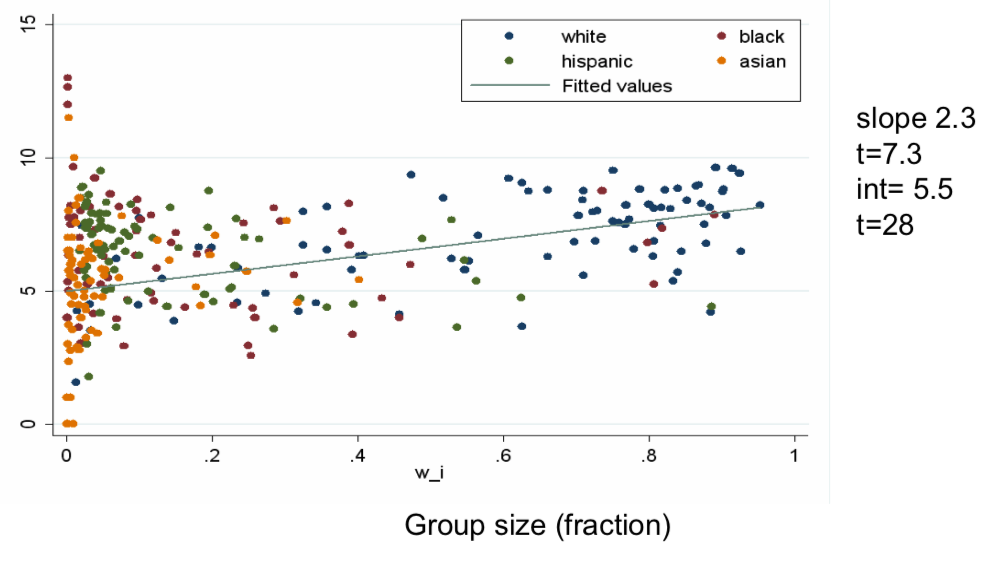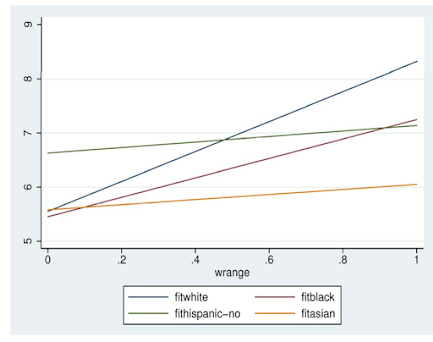# Meeting Process¶

Where do $q_i$ s come from?

Randomness in meetings, but also have $q_i$ s determined by the decisions of the agents

# Meeting Process: "Party"¶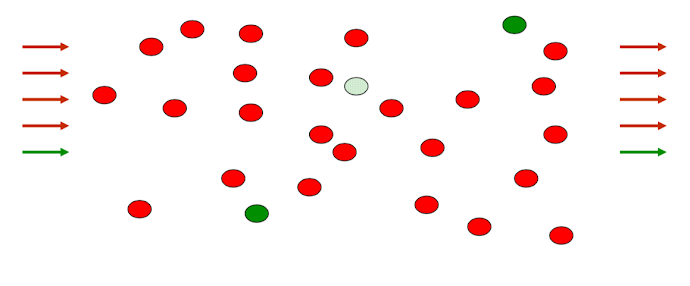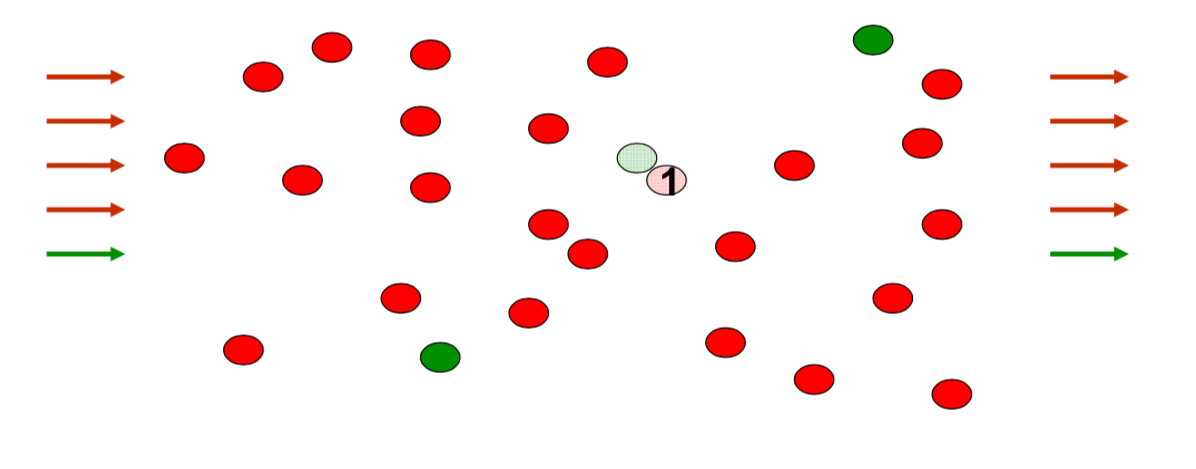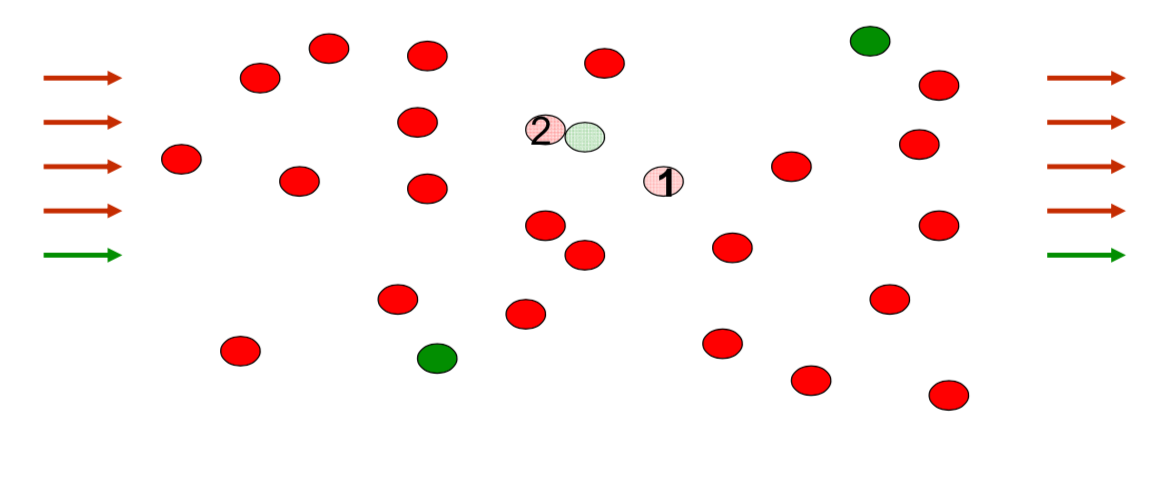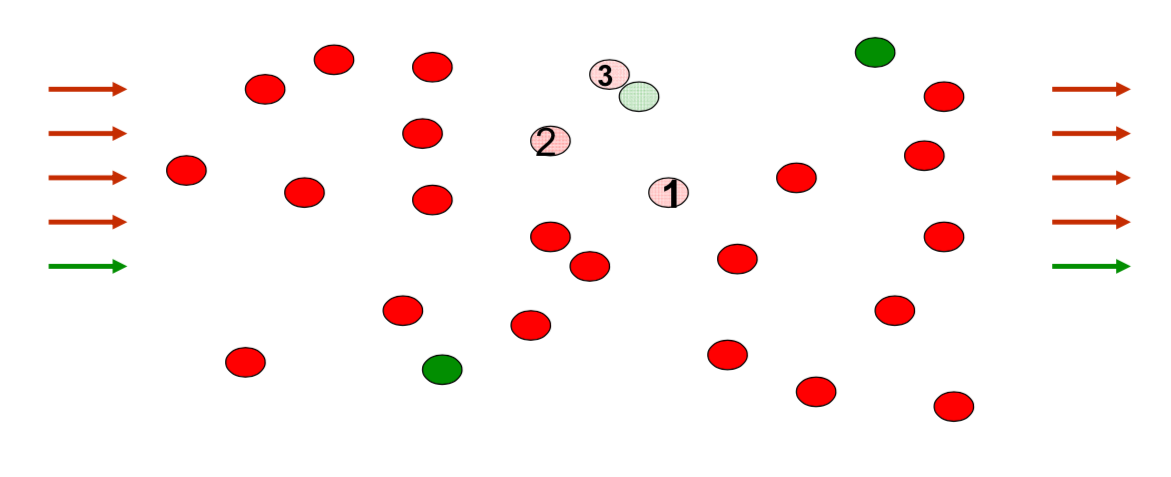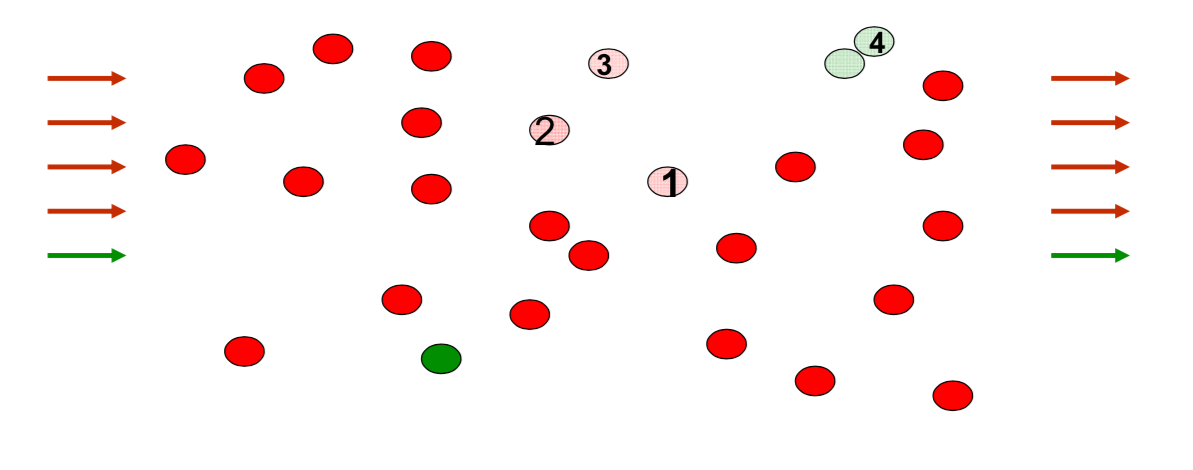# Bias in Meeting Process¶

$q_i$ rate at which type i meets type i $1-q_i$ rate at which type i meets other types

$q_i = (stock_i)^{1/\beta_i}$

$\beta_i = 1$ "unbiased": $q_i = stock_i$

$\beta >1$ meet own types faster than stocks

# Meeting Process¶

$q_i = (stock_i)^{1/\beta_i}$

$beta_i = 1$ if $stock_i = 1/2$ then $q_i = (1/2)^{1/1} = 1/2$

$beta_i = 2$ if $stock_i = 1/2$ then $q_i = (1/2)^{1/2} = 0.707$

$beta_i = 7$ if $stock_i = 1/2$ then $q_i = (1/2)^{1/7} = 0.906$

# Equilibrium Conditions¶

• $t_i$ maximize $(q_it_i + \gamma_i (1-q_i)t_i)^\alpha - ct_i$

• $stock_i = w_i t_i / \sum w_j t_j$ fraction type i in the meeting

• $q_i = (stock_i)^{1/\beta_i}$ meetings determinded by stocks

• $q_i^{\beta_i} = stock_i$ and $\sum stock_i = 1$ imply that $\sum q_i^{\beta_i} = 1$ (balanced meetings)

• atomless population (ignore individual errors)

# Fitting the model¶

• $t_i - \epsilon_i= (\alpha /c)^{1/(1-\alpha)}(q_i + \gamma_i(1 - q_i))^{\alpha/(1-\alpha)}$

• $\sum q_i^{\beta_i} - \epsilon = 1$

# Eliminate (unobserved) costs¶

• $t_i - \epsilon_i= (\alpha /c)^{1/(1-\alpha)}(q_i + \gamma_i(1 - q_i))^{\alpha/(1-\alpha)}$

• $(t_i - \epsilon_i) / (t_j - \epsilon_j) = (q_i + \gamma_i(1 - q_i))^{\alpha/(1-\alpha)} / (q_j + \gamma_j(1 - q_j))^{\alpha/(1-\alpha)}$

$t_i(q_j + \gamma_j(1 - q_j))^{\alpha / (1-\alpha)} - t_j(q_i + \gamma_i(1 - q_i))^{\alpha/(1-\alpha)} = error$

# Fitting Technique¶

Search on grid of biases in preferences and meetings ($\alpha_i, \beta_i, \gamma_i$):

• For each network (school) and specification of biases, calculate an error in terms of total deviation from fitting equations ($t_i, q_i$)

• Sum squared errors across networks (schools)

• Choose biases to minimize (weighted) sum of squared error

# Fitted Values¶